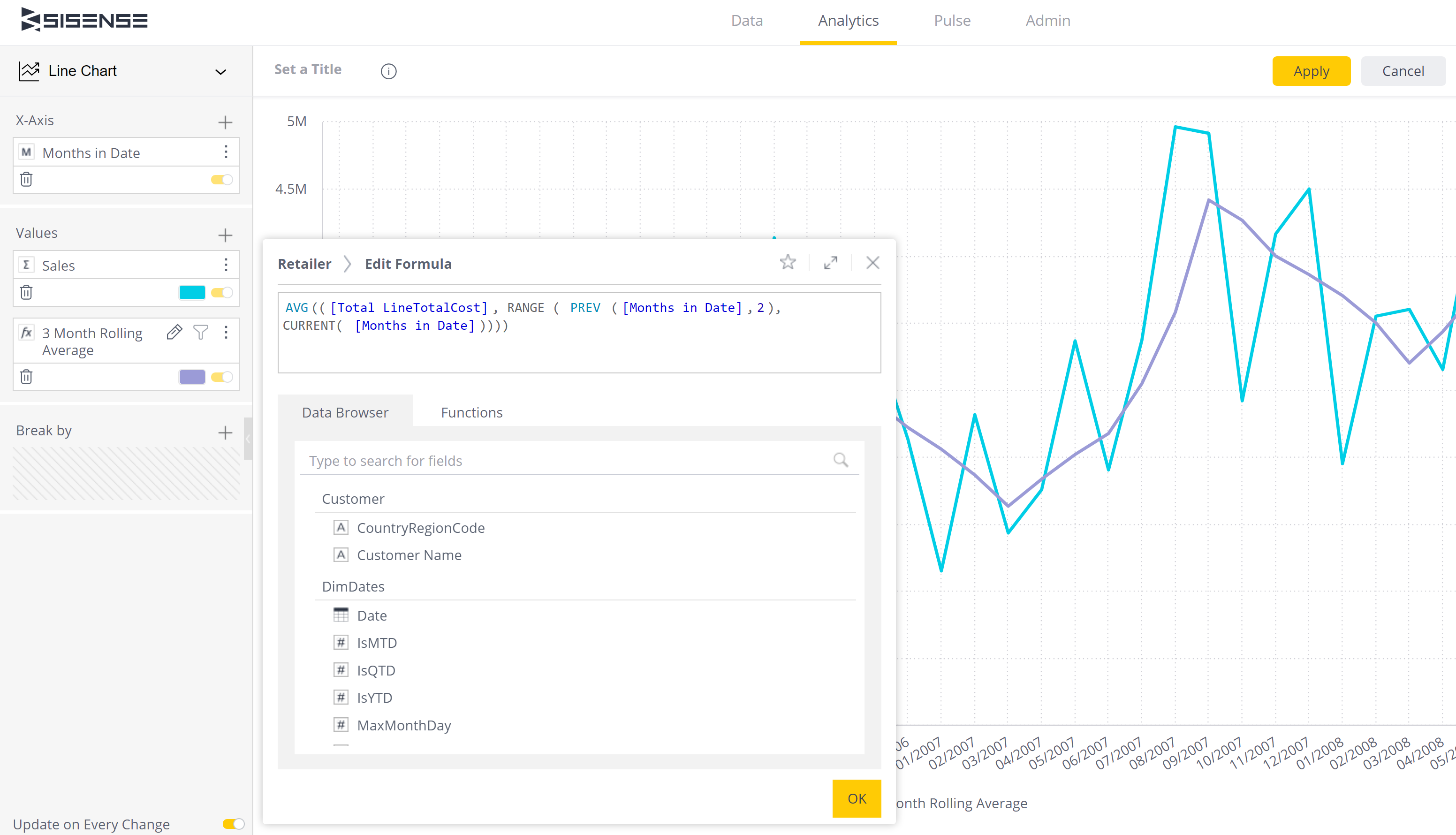Knowledgebase
Knowledgebase : Sisense Tips > Dashboards

Creating a rolling average or sum for a relative date range in a widget in Sisense is a common requirement.The solution is to use a formula using the Range function.

The following assumes [Months in Date] is in the Rows. Where you see [Months in Date] in the formulas below, it is not a filter - just all items.

The basic formula to sum a period of values looks like -

SUM(([Total Sales], RANGE ( PREV ([Months in Date],12), PREV( [Months in Date],1 ))))

This formula sums all the sales from 12 months prior to the relative date to 1 month prior to the relative date (12 months of sales offset by a month from now).

The beauty of this is that it works on the date from the X axis or pivot rather than being relative to now.

This could also be used for a 12 month rolling average by changing this to Average Sales (only change at the front of the formula; if you changed [Total Sales] to [Average Sales] you would be averaging each sale rather than sales for each month).

AVG(([Total Sales], RANGE ( PREV ([Months in Date],12), PREV( [Months in Date],1 ))))

If you wanted to include the current month, you could use the CURRENT function instead of Prev. It would look like -

AVG(([Total Sales], RANGE ( PREV ([Months in Date],11), CURRENT( [Months in Date]))))

Or a 3 month rolling average -

AVG(([Total Sales], RANGE ( PREV ([Months in Date],2), CURRENT( [Months in Date]))))

For any assistance with this contact RAPID BI.